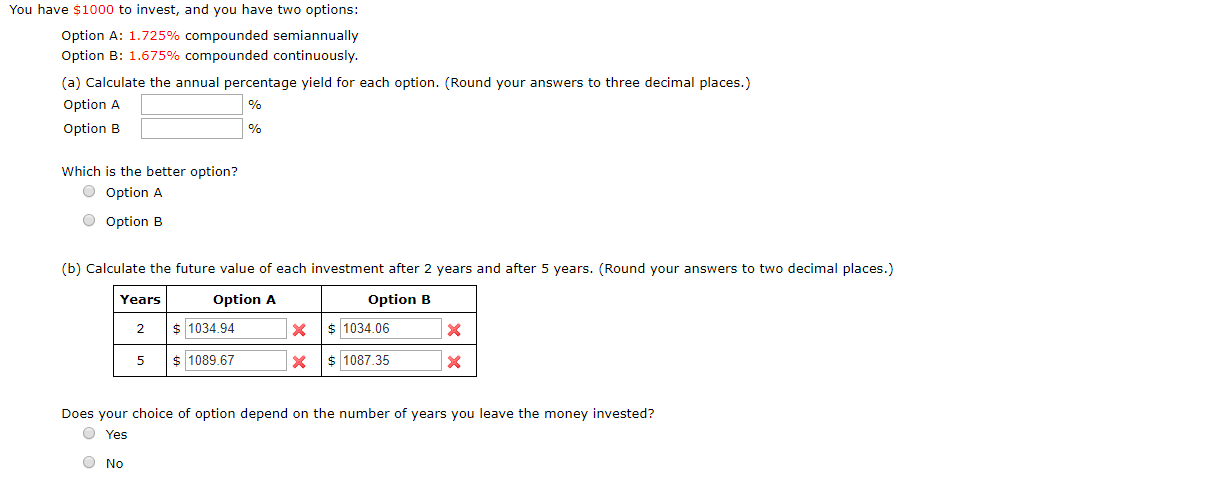# You have \$1000 to invest, and you have two options:Option A: 1.725% compounded semiannuallyOption B: 1.675% compounded continuously.(a) Calculate the annual percentage yield for each option. (Round your answers to three decimal places.)Option AOption B%Which is the better option?O Option AOption B(b) Calculate the future value of each investment after 2 years and after 5 years. (Round your answers to two decimal places.)Option AOption BYears1034.941034.06XX21089.671087.35XXDoes your choice of option depend on the number of years you leave the money invested?Yes

Question
56 viewshelp_outlineImage TranscriptioncloseYou have \$1000 to invest, and you have two options: Option A: 1.725% compounded semiannually Option B: 1.675% compounded continuously. (a) Calculate the annual percentage yield for each option. (Round your answers to three decimal places.) Option A Option B % Which is the better option? O Option A Option B (b) Calculate the future value of each investment after 2 years and after 5 years. (Round your answers to two decimal places.) Option A Option B Years 1034.94 1034.06 X X 2 1089.67 1087.35 X X Does your choice of option depend on the number of years you leave the money invested? Yes fullscreen
check_circle

Step 1

Calculate the annual percentage yield as follows:

Option A:

Step 2

Option B:

Step 3

Option A is b...

### Want to see the full answer?

See Solution

#### Want to see this answer and more?

Solutions are written by subject experts who are available 24/7. Questions are typically answered within 1 hour.*

See Solution
*Response times may vary by subject and question.
Tagged in